# Python中 if 语句及其使用

### 文章目录

• 前言
• 一、 条件测试
• 1.比较字符串相等或不相等
• 2.比较数字
• 3.检查多个条件
• 4.检查特定值是否在列表中
• 二、if 语句
• 1.简单的if语句
• 2. if-else 语句
• 3. if-elif-else 语句
• 三、使用 if 语句处理列表
• 1.使用 if 语句检查列表中的特殊元素
• 2.检查列表是否为空
• 3.使用多个列表
• 总结

• # 前言

本文的主要内容是介绍Python中 if 语句及其使用，包括条件测试、if -else 语句、if -elif-else 语句以及使用 if 语句处理列表操作，文中附有代码以及相应的运行结果辅助理解。

# 一、 条件测试

每条 if 语句的核心都是一个值为True或False的表达式，这种表达式被称为条件测试。Python根据条件测试的值为True还是False来决定是否执行 if 语句中的代码。如果条件测试的值为True，就执行紧跟在 if 语句后面的代码；如果值为False，Python就忽略这些代码。

## 1.比较字符串相等或不相等

下面是条件测试检查变量的值与特定值是否相等/不相等的例子。

``````fruit = 'apple'
print('1.' + str(fruit == 'apple')) #判断是否相等
print('2.' + str(fruit == 'banana'))
print('3.' + str(fruit != 'apple')) #判断是否不相等
print('4.' + str(fruit != 'banana'))
``````

输出结果如下图所示。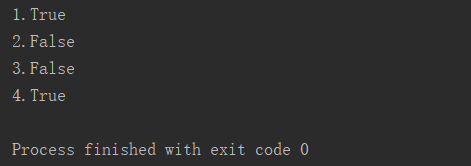由上面的输出结果可知，在比较的过程中，如果与给定的值相等，输出为True，不相等则输出为False。
如果在比较字符串时忽略大小写，可使用函数lower() 将字符串全都转化为小写再比较。

## 2.比较数字

数字的比较与字符串的比较类似，除了相等(==) 和不相等(！=)，还有大于(>)、大于等于 (>=)、小于 (< )和小于等于(<=)。
下面是一个数字比较的例子。

``````num1 = 20
num2 = 30
print('1.' + str(num1 == num2))
print('2.' + str(num1 != num2))
print('3.' + str(num1 >= num2))
print('4.' + str(num1 <= num2))
``````

输出结果如下图所示。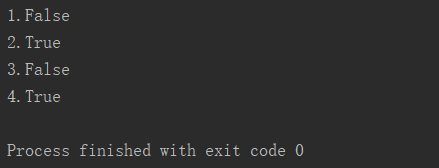## 3.检查多个条件

有时候需要同时检查多个条件，在几个条件都为True时才执行相应的操作，或者只要求多个条件中的一个条件为True时就执行相应的操作，在这些情况下，关键字and和or可以帮助我们轻松实现。
下面是检查多个条件的例子。

``````num1 = 20
num2 = 30
print('1.' + str(num1 >= 15 and num2 >= 15))
print('2.' + str(num1 >= 25 and num2 >= 25))
print('3.' + str(num1 >= 25 or num2 >= 25))
print('4.' + str(num1 >= 35 or num2 >= 35))
``````

输出结果如下图所示。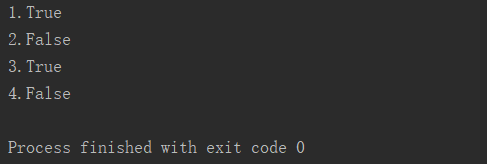## 4.检查特定值是否在列表中

有时候，执行操作前需要检查列表中是否包含特定的值，要判断特定的值是否已包含在列表中，可使用关键字in。
下面是检查特定值是否在列表中的例子。

``````fruits = ['grape', 'apple', 'banana', 'orange', 'pear']
print('1.' + str('apple' in fruits))
print('2.' + str('mango' in fruits))
print('3.' + str('banana' not in fruits))
print('4.' + str('watermelon' not in fruits))
``````

输出结果如下图所示。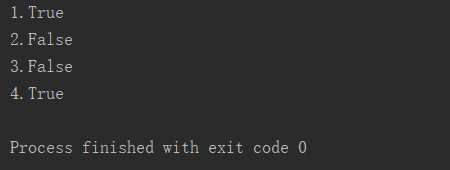# 二、if 语句

弄懂了上面介绍的条件测试后，就可以开始编写if语句了。

## 1.简单的if语句

下面是一个简单的if语句例子。

``````num = 25
if num >= 20:
print('The number is over '+str(num)+'.')
if num < 20:
print('The number is under ' + str(num) + '.')
``````

if语句和for循环类似，在if语句中需要执行的代码要进行缩进。
输出结果如下图所示。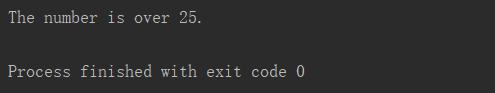## 2. if-else 语句

上面的例子写了两个if语句，其可以由一个if-else 语句代替。

``````num = 25
if num >= 20:
print('The number is over '+str(num)+'.')
else:
print('The number is under ' + str(num) + '.')
``````

输出结果与上面的例子相同。

## 3. if-elif-else 语句

经常需要检查超过两个的情形，为此可使用Python提供的if-elif-else语句，中间的 elif 语句的数量不限，但 if 语句和 else 语句只能各有一个。
下面是 if-elif-else 语句的例子。

``````age = 13
if age <= 3:
print('The age under 3 is free.')
elif age <= 12:
print('The age between 3 and 12 is half price.')
else:
print('The age over 12 is full price.')
``````

输出结果如下图所示。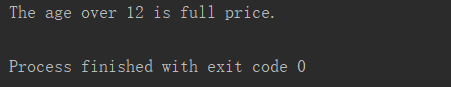# 三、使用 if 语句处理列表

在编程中，结合使用 if 语句和列表可以高效的完成一些任务，当然，与列表配合使用的还有for循环。

## 1.使用 if 语句检查列表中的特殊元素

之所以使用 if 语句检查列表中的特殊元素，是因为这些特殊元素与其他元素对应的输出不同。
下面是使用 if 语句检查列表中的特殊元素的例子。

``````fruits = ['grapes', 'apples', 'bananas', 'oranges', 'pears']
for fruit in fruits:
if fruit == 'apples':
print('Sorry, we are out of apples right now.')
else:
print(fruit.title() + ' are available.')
``````

输出结果如下图所示。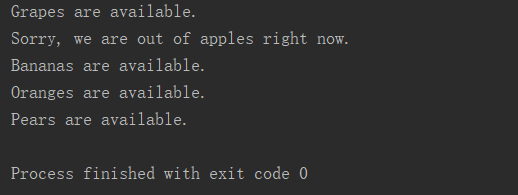由上面的输出结果可以看到，元素apples对应的输出与其他元素的输出不一致，这也正是if 语句的作用所在。

## 2.检查列表是否为空

一般情况下，在操作列表前检查列表是否为空很重要，因为一切操作是基于列表非空进行的。
下面是检查列表是否为空的例子。

``````fruits = []
if fruits:
for fruit in fruits:
if fruit == 'apples':
print('Sorry, we are out of apples right now.')
else:
print(fruit.title() + ' are available.')
else:
print('The list is empty.')
``````

输出结果如下图所示。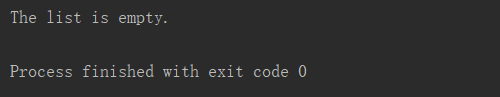## 3.使用多个列表

我们买东西时会自己先列一个清单，然后商店也有自己的货物清单，当你清单上的商品也在商店的货物清单上，你可以购得该商品，否则不行。
下面是一个使用多个列表进行操作的例子。

``````fruits_available = ['grapes', 'apples', 'bananas', 'oranges', 'pears']
fruits_want = ['mangoes', 'apples', 'bananas', 'watermelons', 'pears']
for fruit in fruits_want:
if fruit in fruits_available:
print('We have ' + fruit +'.')
else:
print("Sorry, we don't have " + fruit +'.')
print('Check complete!')
``````

输出结果如下图所示。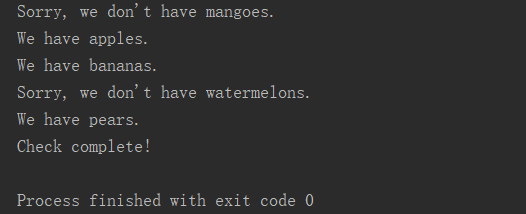由输出结果，我们就可以知道哪些商品自己在该店铺内可以买到，哪些买不到。

# 总结

以上就是Python中 if 语句及其使用的所有内容了，应当熟悉 if 语句、 if-else 语句、 if-elif-else 语句分别适用于哪种场合，同时也应当学会 if 语句、for循环以及列表的综合操作。
本文参考书目：Python 编程 ：从入门到实践 / (美) 埃里克•马瑟斯(Eric Matthes)著；袁国忠译

来源：西岸贤

物联沃分享整理
物联沃-IOTWORD物联网 » Python中 if 语句及其使用